# Class 12 Maths NCERT Solutions for Chapter 7 Integrals Miscellaneous Exercise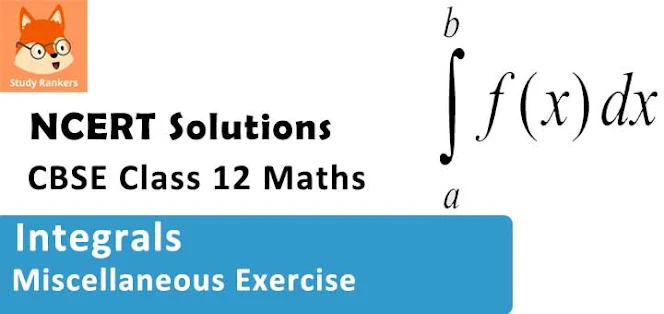### Integrals Exercise Miscellaneous Solutions

1. Integrate the function 1/(x – x3)

Solution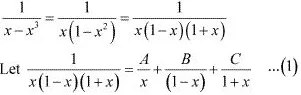⇒1 = A(1 - x2 ) + Bx(1 + x) + Cx(1 - x)
⇒ 1 = A - Ax2 + Bx + Bx2 + Cx - Cx2
Equating the coefficients of x2 , x, and constant term, we obtain
-A + B - C = 0
B + C = 0
A = 1
On solving these equations, we obtain
A = 1, B = 1/2, and C = -1/2
From equation (1), we obtain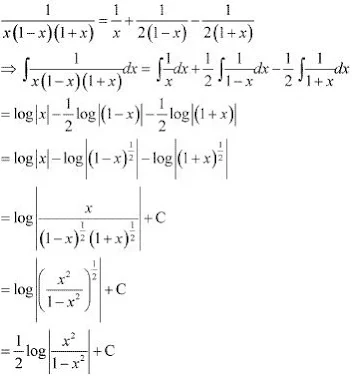2. Integrate the functions 1/[(√x + a) + √(x + b)]
Solution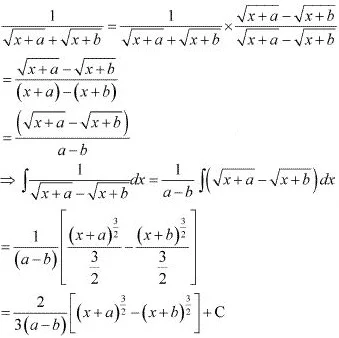3. Integrate the functions 1/x√(ax - x2) [Hint: Put x = a/t]
Solution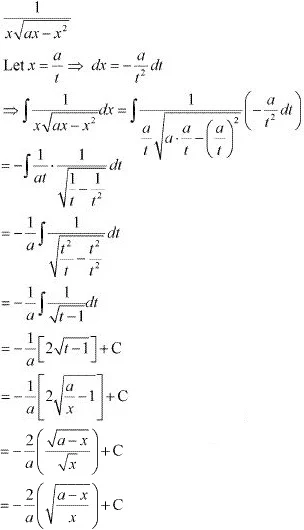4. Integrate the functions 1/[x2 (x4 + 1)3/4]
Solution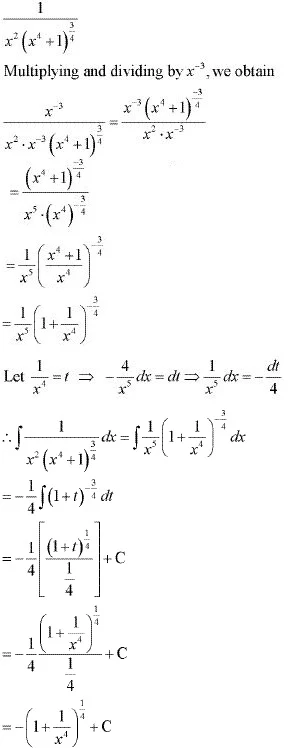5. Integrate the functions 1/(x1/2 + x1/3) [ Hint: 1/(x1/2 + x1/3) = 1/[x1/3 (1 + x1/6) put x = t6
Solution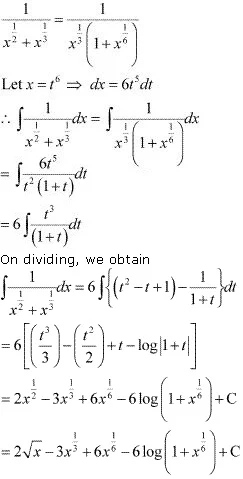6. Integrate the functions 5x/[(x + 1)(x2 + 9)].
Solution⇒ 5x = A(x2 + 9) + (Bx + C)(x + 1)
⇒ 5x = Ax2 + 9A + Bx2 + Bx + Cx + C
Equating the coefficients of x2 , x, and constant term, we obtain
A + B = 0
B + C = 5
9A + C = 0
On solving these equations, we obtain
a = -1/2, B = 1/2 , and C = 9/2
From equation (1), we obtain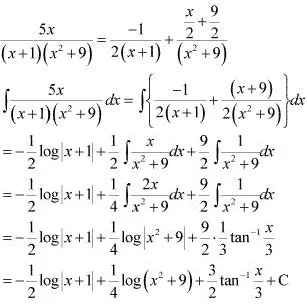7. Integrate the functions sin x/sin(x - a)
Solution
sin x/sin(x - a)
Let x - a = t ⇒ dx = dt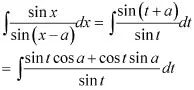= ∫(cos a + cot t sin a) dt
= t cos a + sin a log |sin t| + C1
= (x - a) cos a + sin a log |sin (x - a)| + C1
= x cos a + sin a log |sin(x - a)| - a cos a + C1
= sin a log |sin(x - a)| + x cos a + C

8. Integrate the functions (e5log x - e4log x)/(e3log x - e2log x)
Solution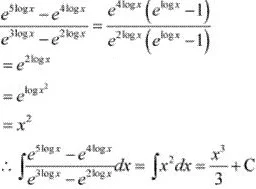9. Integrate the functions cos x/√(4 - sin2 x)
Solution
cos x/√(4 - sin2 x)
Let sin x = t ⇒ cos x dx = dt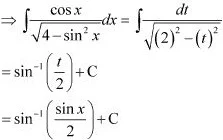10. Integrate the functions (sin8x - cos8x)/(1 - 2sin2x cos2x)
Solution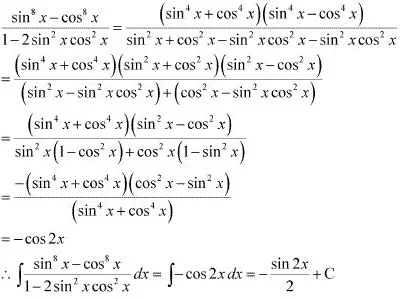11. Integrate the functions 1/[cos(x + a) cos(x + b)]
Solution
1/[cos(x + a) cos(x + b)]
Multiplying and dividing by sin(a - b), we obtain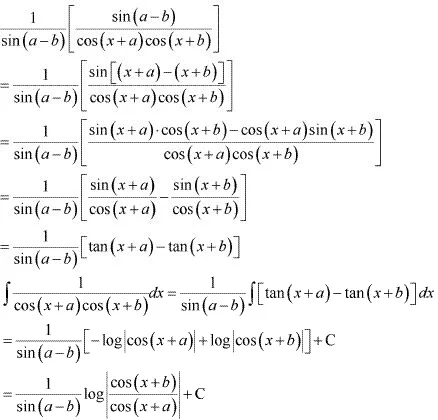12. Integrate the functions x3/√(1 - x8)
Solution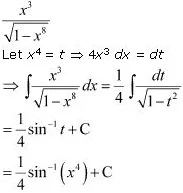13. Integrate the functions ex/[(1 + ex)(2 + ex)].
Solution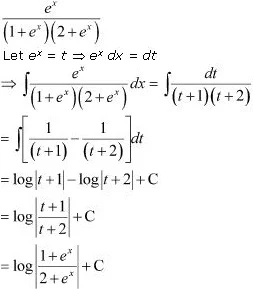14. Integrate the functions 1/[(x2 + 1)(x2 + 4)]
Solution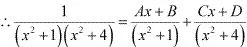⇒ 1 = (Ax + B)(x2 + 4) + (Cx + D)(x2 + 1)
⇒ 1 = Ax3 + 4Ax + Bx2 + 4B + Cx3 + Cx + Dx2 + D
Equating the coefficients of x3 , x2 , x, and constant term, we obtain
A + C = 0
B + D = 0
4A + C = 0
4B + D = 1
On solving these equations, we obtain
A = 0, B = 1/3, C = 0, and D = -1/3
From equation (1), we obtain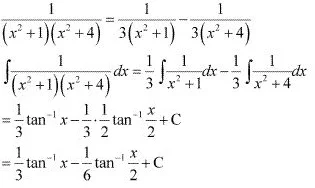15.  Integrate the functions cos3 xelog sinx
Solution
cos3 xe log sinx = cos3 x × sin x
Let cos x = t ⇒ - sin x dx = dt
= -∫t3 dt
= (-t4/4) + C
= (-cos4 x/4)  + C

16. Integrate the functions e3log x  (x4 + 1)-1
Solution
e3log x (x4 + 1)-1 = elog x3 (x4 + 1)-1 = x3 /(x4 + 1)
Let x4 + 1 = t ⇒ 4x3 dx = dt
⇒ ∫elog x (x4 + 1)-1 dx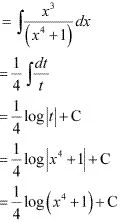17. Integrate the functions f'(ax + b)[f(ax + b)]n
Solution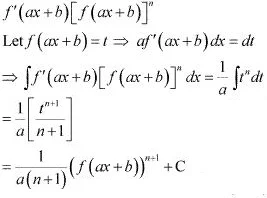18. Integrate the functions 1/√[sin3 x sin (x + a)]
Solution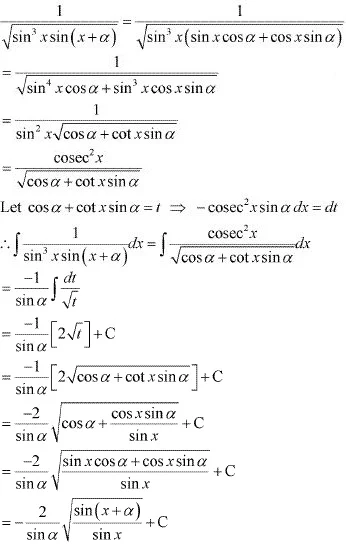19. Integrate the functions (sin-1 √x - cos-1 √x)/(sin-1√x + cos-1√x) , x ∊ [0, 1]
Solution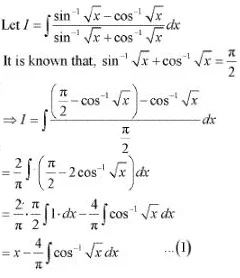Let I1 = ∫cos-1 √x dx
Also, let √x = t ⇒ dx = 2t dt
⇒ I1 = 2∫cos-1 t.t dt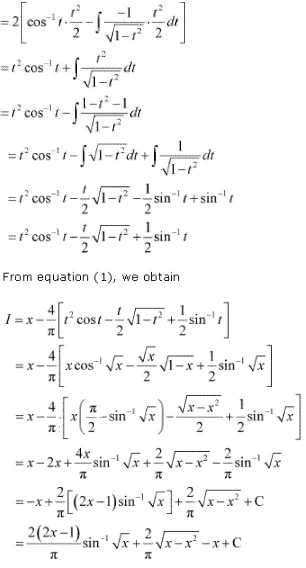20 Integrate the functions √[(1 - √x)/(1 + √x)]
Solution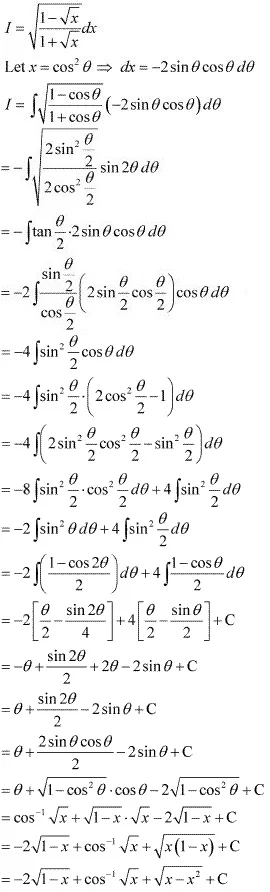21. Integrate the functions [(2 + sin 2x)/(1 + cos 2x)] ex    .
Solution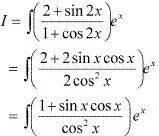= ∫(sec2 x + tan x)ex
let f(x) = tan x ⇒ f'(x) = sec2 x
∴ I = ∫[f(x) + f'(x)]ex dx
= ex f(x) + C
= ex tan x + C

22. Integrate the functions (x2 + x + 1)/[(x + 1)2 (x +  2)]
Solution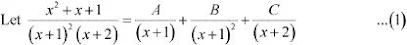⇒ x2 + x + 1 = A(x + 1)(x + 2) + B(x + 2) + C(x2 + 2x + 1)
⇒ x2 + x + 1 = A(x2 + 3x + 2) + B(x + 2) + C(x2 + 2x + 1)
⇒ x2 + x + 1 = (A + C)x2 + (3A + B + 2C)x + (2A + 2B + C)
Equating the coefficients of x2, x, and constant term, we obtain
A + C = 1
3A + B + 2C = 1
2A + 2B + C = 1
On solving these equations, we obtain
A = -2, B = 1, and C = 3
From equation (1), we obtain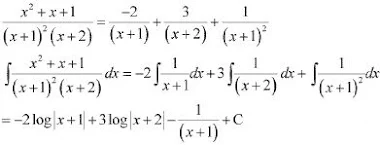23 Integrate the functions tan-1 √[(1 - x)/(1 + x)]
Solution
I =  tan-1 √[(1 - x)/(1 + x)]  dx
Let x = cos θ ⇒ dx = - sin θ dθ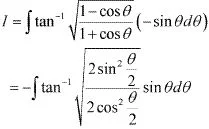= - ∫ tan-1 tan(θ/2) . sin θ dθ
= -(1/2)∫ θ. sin θ dθ
= -(1/2)[θ . (- cosθ) - ∫1.(- cosθ)dθ]
= -(1/2)[-θ cos θ + sin θ]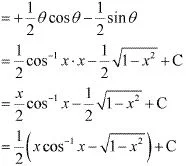24. Integrate the functions [√x2 + 1{log(x2 + 1) - 2 log x}]/x4.
Solution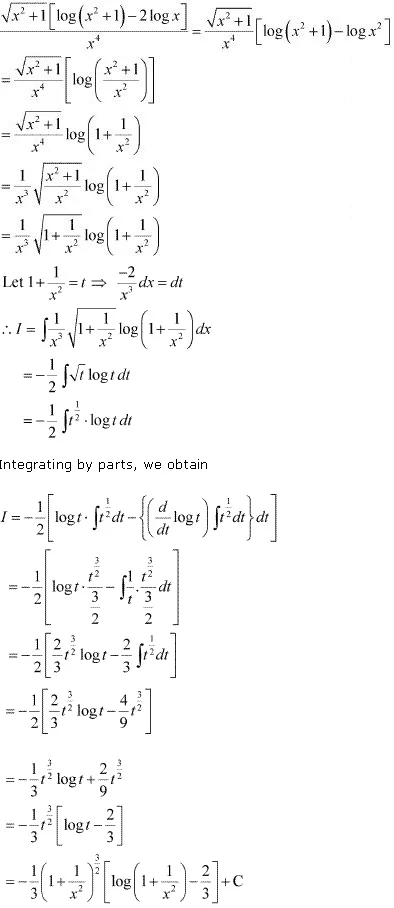25. Evaluate the definite integrals  ∫π/2π  ex (1 - sin x)/(1 - cos x)] dx
Solution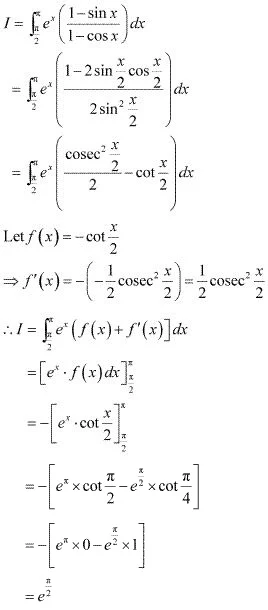26. Evaluate the definite integrals ∫0π/4 [(sin x cos x)/(cos4 x + sin4 x)] dx
Solution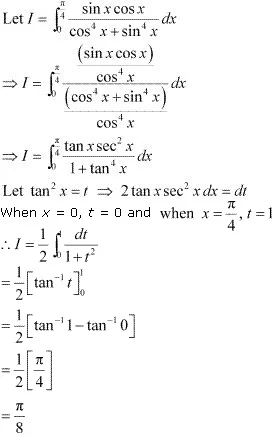27. Evaluate the definite integrals ∫0π/2 (cos2 x dx)/[(x cos2 x + 4 sin2 x)]
Solution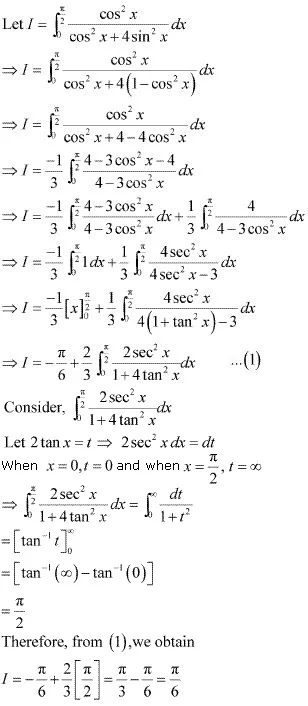28. Evaluate the definite integrals ∫π/6 π/3 [(sin x + cos x)/√(sin2x)] dx
Solution29. Evaluate the definite integrals ∫01 dx/[√(1 + x) - √x]
Solution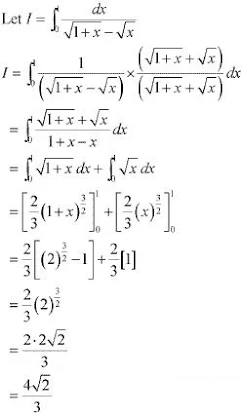30. Evaluate the definite integrals ∫0π/4 [(sin x + cos x)/(9 + 16 sin 2x)] dx
Solution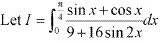Also, let sin x - cos x = t ⇒ (cos x + sin x)dx = dt
When x = 0, t = -1 and when x = π/4, t = 0
⇒ (sin x - cos x)2 = t2
⇒ sin2 x + cos2 x  - 2sin x cos x = t2
⇒ 1 - sin 2x = t2
⇒ sin 2x = 1 - t2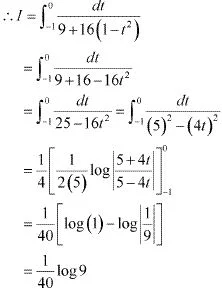31. Evaluate the definite integrals ∫0π/2 sin 2x tan-1 (sin x) dx
Solution
Let I =  ∫0π/2 sin 2x tan-1 (sin x) dx =  ∫0π/2 2sin x cos x tan-1 (sin x) dx
Also, let sin x = t ⇒ cos x dx = dt
When x = 0, t = 0 and when x = π/2, t = 1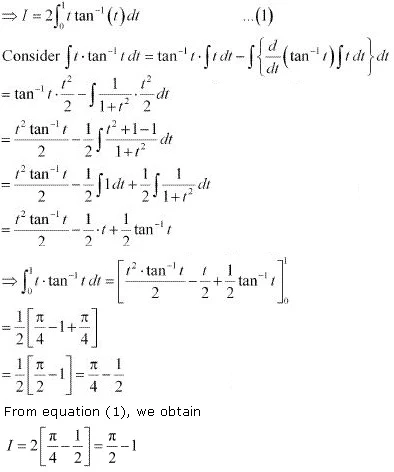32. Evaluate the definite integrals ∫0π [(x tan x)/(sec x + tan x)] dx
Solution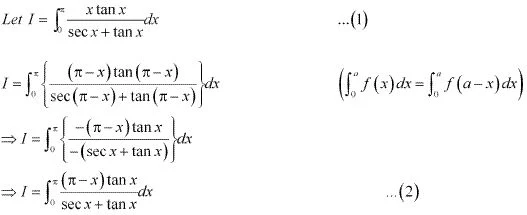Adding (1) and (2), we obtain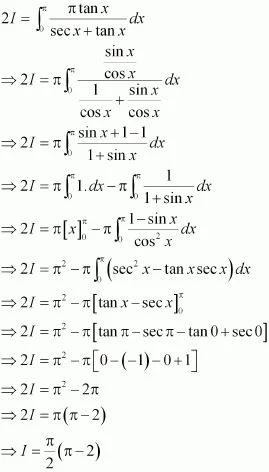33. Evaluate the definite integrals ∫14 [|x -1| + |x - 2| + |x - 3|] dx
Solution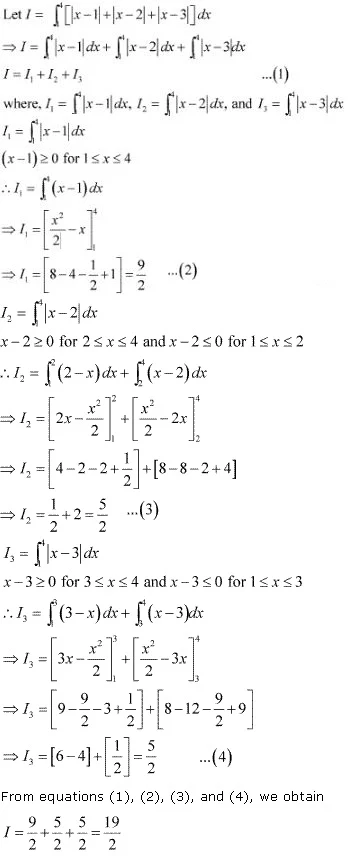34. Prove the following ∫13 dx/[x2 (x + 1)] = 2/3 + (log 2)/3
Solution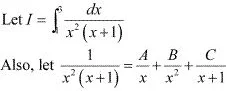⇒ 1 = Ax(x + 1) + B(x + 1) + C(x2 )
⇒ 1 = Ax2 + Ax + Bx + B + Cx2
Equating the coefficients of x2 , x, and constant term, we obtain
A + C = 0
A + B = 0
B = 1
On solving these equations, we obtain
A = -1, C = 1, and B = 1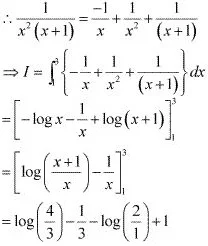= log 4 + log 3 - log 2 + 2/3
= log 2 - log 3 + 2/3
= log(2/3) + 2/3
Hence, the given result is proved.

35. Prove the following  ∫04  xex dx = 1
Solution
Let I = ∫04  xex dx
Integrating by parts, we obtain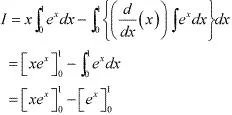= e- e + 1
= 1
Hence, the given result is proved .

36. Prove the following ∫-11 x17 cos4 x dx = 0
Solution
Let I =  ∫-11 x17 cos4 x dx
Also, let f(x) = x17 cos4 x
⇒ f(-x) = (-)17 cos4 (-x) = -x17 cos4 x = - f(x)
Therefore, f(x) is an odd function.
It is known that if f(x) is an odd function, then ∫-11  f(x) dx = 0
∴ I = ∫-11 x17 cos4 x dx = 0
Hence, the given result is proved.

37. Prove the following  ∫0π/2 sin3 x dx = 2/3
Solution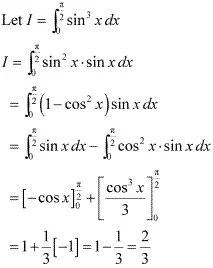Hence, the given result is proved .

38. Prove the following ∫0π/4 2 tan3 x dx = 1 - log 2
Solution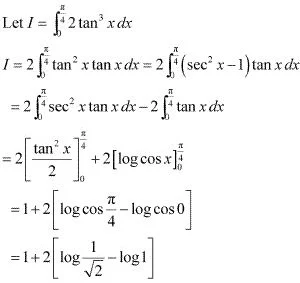= 1 - log 2 - log 1 = 1 - log 2
Hence, the given result is proved.

39. Prove the following  ∫01 sin-1 x dx = π/2 - 1
Solution
Let I = ∫01 sin-1 x dx
⇒ I = ∫01 sin-1 x.1. dx
Integrating by parts, we obtain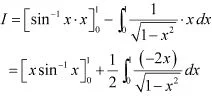Let 1 - x3 = t ⇒ -2x dx = dt
When x = 0, t = 1 and when x = 1, t = 0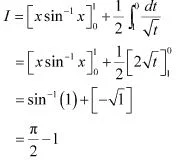Hence, the given result is proved.

40. Evaluate ∫01 e2-3x dx as a limit of a sum.
Solution
Let I = ∫01 e2-3x dx
It is known that,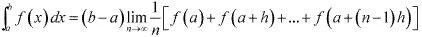Where,  h = (b - a)/n
Here, a = 0, b = 1, and f(x) = e2-3x
⇒ h = (1 - 0)/n = 1/n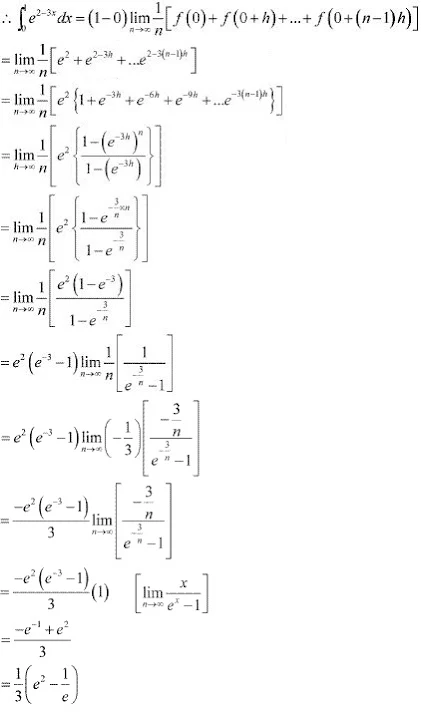41. Choose the correct ∫[dx/(ex + e - x)] is equal to
(A) tan-1 (ex ) + C
(B) tan-1 (e-x ) + C
(C) log(ex - e-x ) + C
(D) log(ex + e-x ) + C
Solution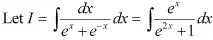Also, let ex = t ⇒ ex dx = dt
∴ I = ∫[dt/(1 + t2 )]
= tan-1 t + C
= tan-1 (ex ) + C
Hence, the correct answer is A.

42. Choose the correct answers ∫[cos 2x/(sin x + cos x)2] dx is equal to
(A). -1/(sin x + cos x) + C
(B) log |sin x + cos x| + C
(C) log |sin x + cos x| + C
(D) 1/(sin x - cos x)2
Solution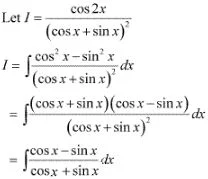Let cos x + sin x = t ⇒ ( cos x - sin x)dx = dt
∴ I = ∫ dt/t
= log|t| + C
= log | cos x + sin x| + C
Hence, the correct answer is B.

43. Choose the correct answers If f (a + b - x) = f (x), then ∫ab x f(x) dx is equal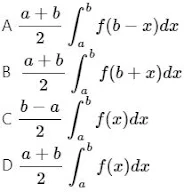Solution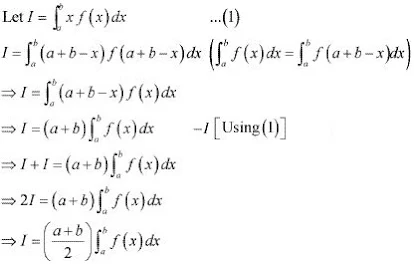Hence, the correct answer is D.

44. Choose the correct answer The value of ∫01 tan-1(2x - 1)/(1 + x - x2) dx is
(A) 1
(B) 0
(C) -1
(D) π/4
Solution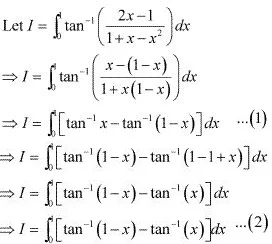Adding (1) and (2), we obtain
2I = ∫01 (tan-1 x + tan-1 (1 - x) - tan-1 (1 - x) - tan-1 x) dx
⇒ 2I = 0
⇒ I = 0
Hence, the correct answer is B.